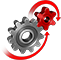# WolframSystemModeler

## Thermo–Fluid Systems: Entropy Transfer

Addition of heat can lead to flow of fluid from a high entropy to a low entropy region. In this example, we see how solar radiation can lead to fluid flow between a storage tank and a tanker.

### Model of the Tank System

The storage tank is connected to a tanker via a non return valve and a pipe. Heat is added to the storage tank by means of solar radiation.Storage tank connected to a tanker.

### Compare Mass Flow for Different Fluids

The mass flow rate of natural gas is about 25 times more than that of water for the same amount of solar radiation.
The red curve in the top plot shows the amount of heat added by solar radiation. The blue curve in the middle plot shows the mass flow rate for water. The orange curve in the last plot shows the mass flow rate for natural gas.

### Simulate Delays Due to Pipe Lengths

As the pipe length increases a delay is introduced between the peak of the solar radiation and peak of the mass flow rate.The plot shows mass flow rates for different pipe lengths. Natural gas is used as the working fluid.

Model thermo-fluid systems

## Wolfram System Modeler

Questions? Comments? Contact a Wolfram expert »